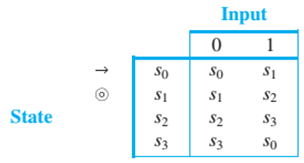Chapter 12.2, Problem 9ES### Discrete Mathematics With Applicat...

5th Edition
EPP + 1 other
ISBN: 9781337694193

#### Solutions

Chapter
Section### Discrete Mathematics With Applicat...

5th Edition
EPP + 1 other
ISBN: 9781337694193
Textbook Problem
1 views

# In 8 and 9, a finite-state automaton is given by an annotated next-state table. For each automaton: a. Find its states. b. Find its input symbols. c. Find its initial state. d. Find its accepting states. e. Draw its transition diagram. 9. Next-State TableTo determine

(a)

Find its states.

Explanation

Given information:

Concept used:

The states of the automaton are represented by first column of the annotated table

Calculation:

The states of the automaton are represented by first column of the annotated table...

To determine

(b)

Find its input symbol.

To determine

(c)

Find its initial state.

To determine

(d)

Find its accepting states.

To determine

(e)

Find its transition diagram.

### Still sussing out bartleby?

Check out a sample textbook solution.

See a sample solution

#### The Solution to Your Study Problems

Bartleby provides explanations to thousands of textbook problems written by our experts, many with advanced degrees!

Get Started